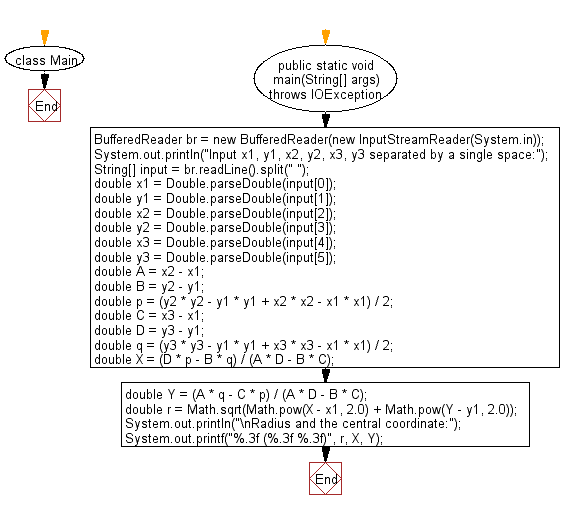# Java Exercises: Compute the radius and the central coordinate of a circle

## Java Basic: Exercise-218 with Solution

Write a Java program to compute the radius and the central coordinate (x, y) of a circle which is constructed by three given points on the plane surface.

Input:

x1,y1,x2,y2,x3,y3,xp,yp separated by a single space.

Sample Solution:

Java Code:

`````` import java.io.IOException;

class Main {
public static void main(String[] args) throws IOException {
System.out.println("Input x1, y1, x2, y2, x3, y3 separated by a single space:");

double x1 = Double.parseDouble(input);
double y1 = Double.parseDouble(input);
double x2 = Double.parseDouble(input);
double y2 = Double.parseDouble(input);
double x3 = Double.parseDouble(input);
double y3 = Double.parseDouble(input);

double A = x2 - x1;
double B = y2 - y1;
double p = (y2 * y2 - y1 * y1 + x2 * x2 - x1 * x1) / 2;
double C = x3 - x1;
double D = y3 - y1;
double q = (y3 * y3 - y1 * y1 + x3 * x3 - x1 * x1) / 2;

double X = (D * p - B * q) / (A * D - B * C);
double Y = (A * q - C * p) / (A * D - B * C);

double r = Math.sqrt(Math.pow(X - x1, 2.0) + Math.pow(Y - y1, 2.0));
System.out.printf("%.3f (%.3f %.3f)", r, X, Y);
}
}
```
```

Sample Output:

```Input x1, y1, x2, y2, x3, y3 separated by a single space:
5 6 4 8 7 9

1.821 (5.786 7.643)
```

Flowchart:Java Code Editor:

What is the difficulty level of this exercise?

﻿

## Java: Tips of the Day

Parsing dates:

```import java.io.*;
import java.util.*;
import java.text.*;

String s = "2001/09/23 14:39";

SimpleDateFormat formatter = new SimpleDateFormat ("yyyy/MM/dd H:mm");
Date d = formatter.parse(s, new ParsePosition(0));
```